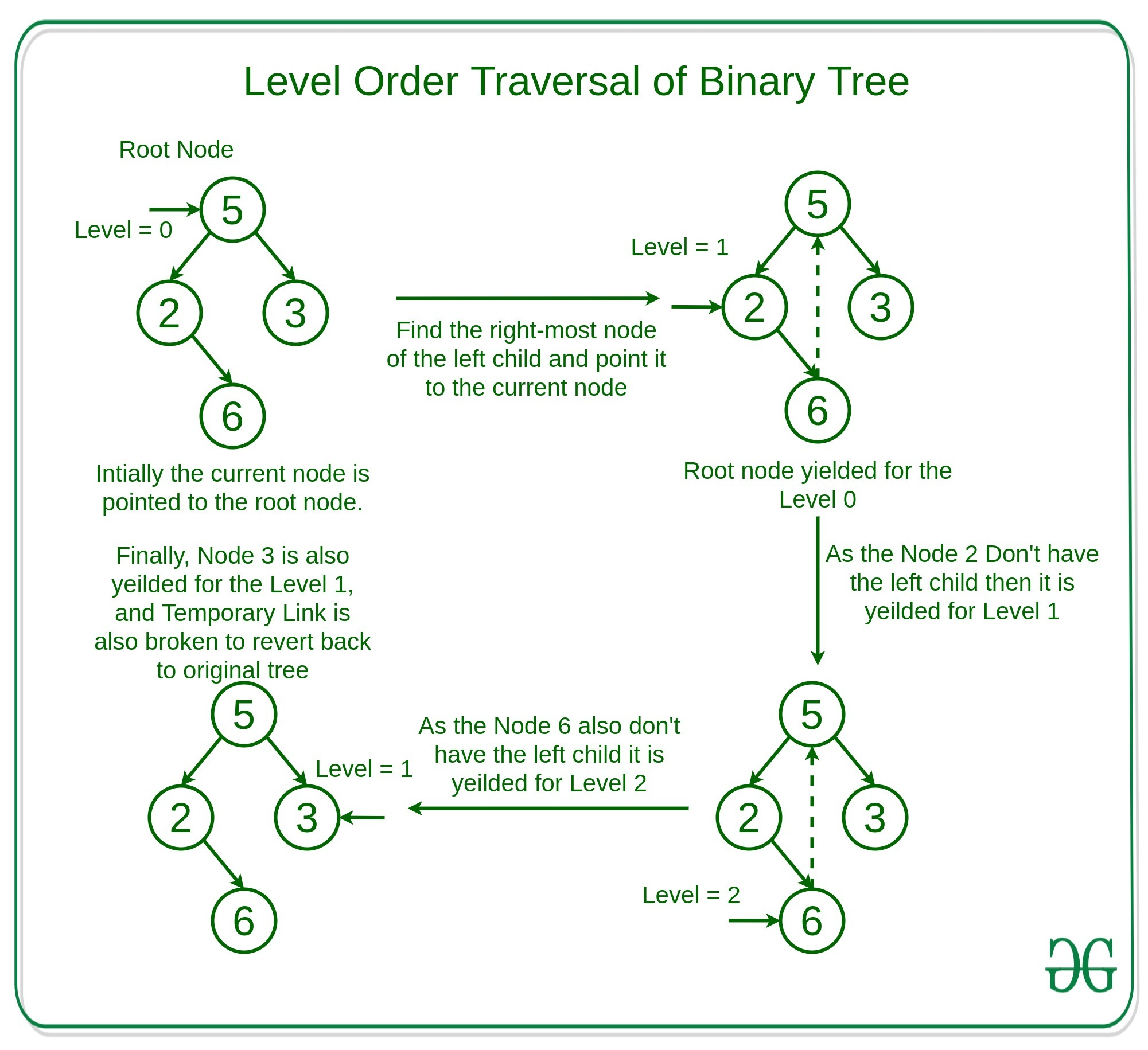# Level order traversal of Binary Tree using Morris Traversal

• Difficulty Level : Medium
• Last Updated : 29 Dec, 2021

Given a binary tree, the task is to traverse the Binary Tree in level order fashion.
Examples:

```Input:
1
/ \
2   3
Output:
1
2 3

Input:
5
/ \
2   3
\
6
Output:
5
2 3
6```

Approach: The idea is to use Morris Preorder Traversal to traverse the tree in level order traversal.
Observations: There are mainly two observations for the traversal of the tree using Morris preorder traversal. That is –

• In Preorder traversal the left-most nodes of a level are visited first due which it can be used to traverse the tree in level order fashion.
• As we maintain the horizontal distance of the nodes in the top view of the binary tree, Similarly If we maintain the level of the current node and increment or decrement the level accordingly as per the movement, Then the nodes can be traversed easily.

As in the Morris preorder traversal, we connect the right-most node of the left child to its inorder successor to maintain the movement such that we can traverse back to the right child of the parent node after completely exploring the left child of the parent. Therefore, while moving to the rightmost child of the left child we can keep track of the number of the increment in the level to compute the level inorder successor of the child.
Below is the explanation of the approach with the help of example:Below is the implementation of the above approach:

## Python3

 `# Python implementation of the Level ` `# order traversal using Morris traversal`   `# Class of the node of the ` `# Binary Tree` `class` `Node:` `    ``def` `__init__(``self``, data):` `        ``self``.data ``=` `data ` `        ``self``.left ``=` `None` `        ``self``.right ``=` `None`   `# Function to traverse the Binary` `# tree in the Level Order Fashion` `def` `levelOrderTraversal(root):` `    `  `    ``# Current Node is marked as` `    ``# the Root Node` `    ``curr ``=` `root` `    ``level ``=` `0`   `    ``# Loop to traverse the Binary` `    ``# Tree until the current node ` `    ``# is not Null` `    ``while` `curr:`   `        ``# If left child is null, print the ` `        ``# current node data. And, update  ` `        ``# the current pointer to right child. ` `        ``if` `curr.left ``is` `None``:`   `            ``# Return the current node with` `            ``# its level` `            ``yield` `[curr, level] ` `            ``curr ``=` `curr.right` `            ``if` `curr:` `                ``level ``+``=` `1` `            ``else``:` `                ``level ``-``=` `1` `        ``else``:`   `            ``# Find the inorder predecessor ` `            ``prev ``=` `curr.left ` `            ``to_up ``=` `0`   `            ``# Loop to find the right most ` `            ``# node of the left child of the` `            ``# current node` `            ``while` `prev.right ``is` `not` `None` `and` `\` `                    ``prev.right ``is` `not` `curr: ` `                ``prev ``=` `prev.right` `                ``to_up ``+``=` `1`   `            ``# If the right child of inorder ` `            ``# predecessor already points to ` `            ``# the current node, update the  ` `            ``# current with it's right child ` `            ``if` `prev.right ``is` `curr:` `                ``prev.right ``=` `None` `                ``curr ``=` `curr.right ` `                ``level ``-``=` `to_up ``+` `1` `            `  `            ``# else If right child doesn't` `            ``# point to the current node, ` `            ``# then print this node's data ` `            ``# and update the right child` `            ``# pointer with the current node  ` `            ``# and update the current with` `            ``# it's left child ` `            ``else``:` `                ``yield` `[curr, level]` `                ``prev.right ``=` `curr  ` `                ``curr ``=` `curr.left` `                ``level ``+``=` `1`   `# Driver Code` `if` `__name__ ``=``=` `"__main__"``:` `    ``root ``=` `Node(``5``)` `    ``root.left ``=` `Node(``2``)` `    ``root.right ``=` `Node(``3``)` `    ``root.left.right ``=` `Node(``6``)`   `    ``# Output List to store the ` `    ``# Level Order Traversed Nodes` `    ``outputData ``=` `[[] ``for` `i ``in` `range``(``100``)]`   `    ``for` `node, level ``in` `levelOrderTraversal(root):` `        ``outputData[level].append(node.data)`   `    ``h ``=` `0`   `    ``# Loop to find the height of the ` `    ``# Binary Tree` `    ``for` `i ``in` `outputData:` `        ``if` `i:` `            ``h ``+``=` `1` `        ``else``:` `            ``break`   `    ``# Loop to print the Data` `    ``for` `i ``in` `range``(h):` `        ``print``(``' '``.join(``map``(``str``, outputData[i])))`

Output:

```5
2 3
6```

Performance Analysis:

• Time Complexity: As in the above approach, every node is touched at max twice due to which the time complexity is O(N), where N is the number of nodes.
• Auxiliary Space: As in the above approach, there is no extra space used due to which auxiliary space used will be O(1)

My Personal Notes arrow_drop_up
Recommended Articles
Page :